•Fresh & Alive
•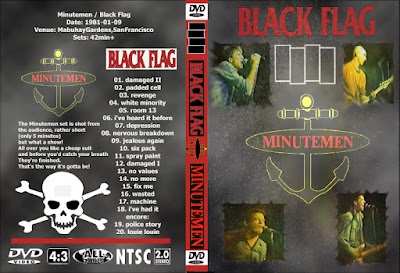T.U.B.E.
•T.U.B.E.
•T.U.B.E.
•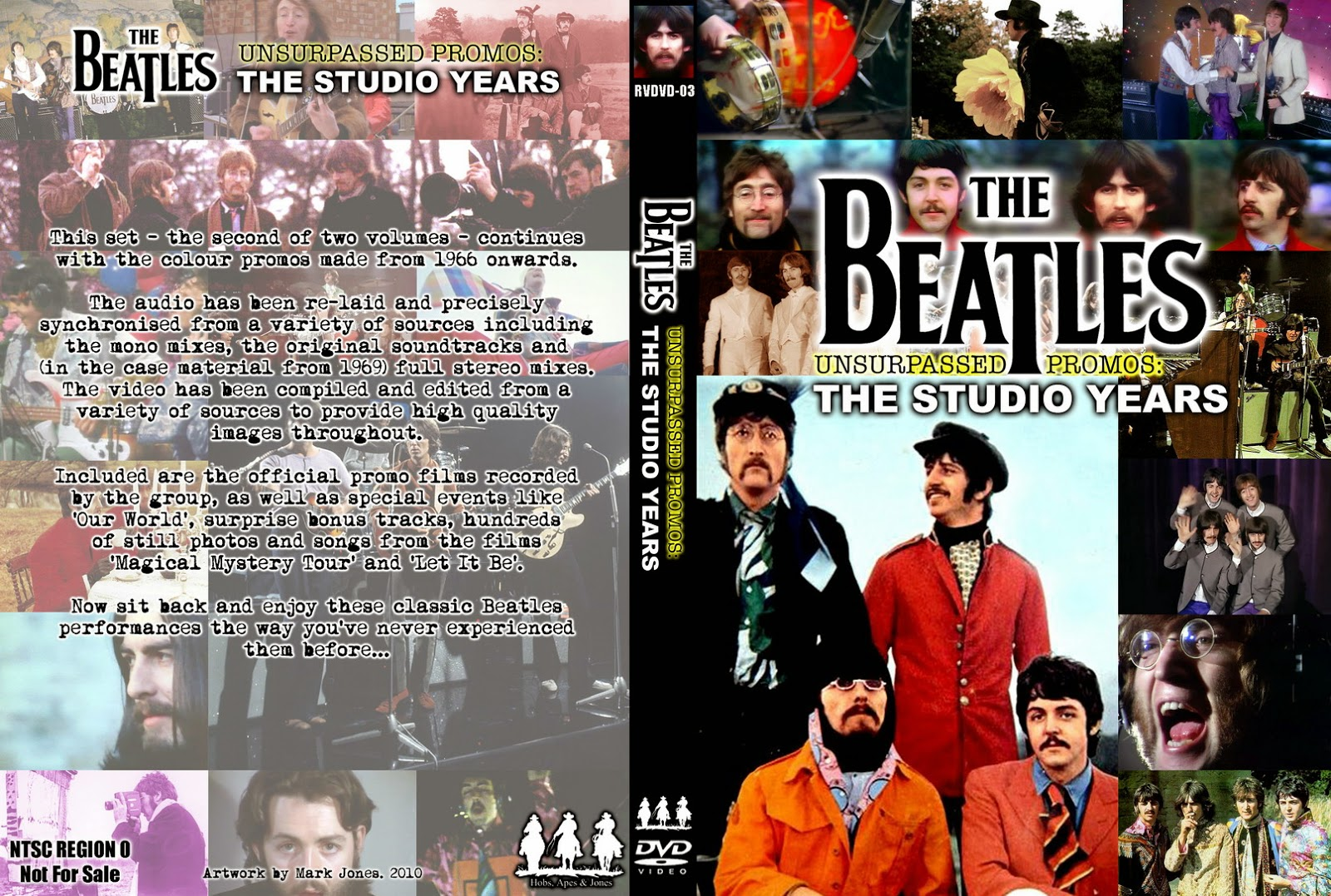T.U.B.E.
•T.U.B.E.
•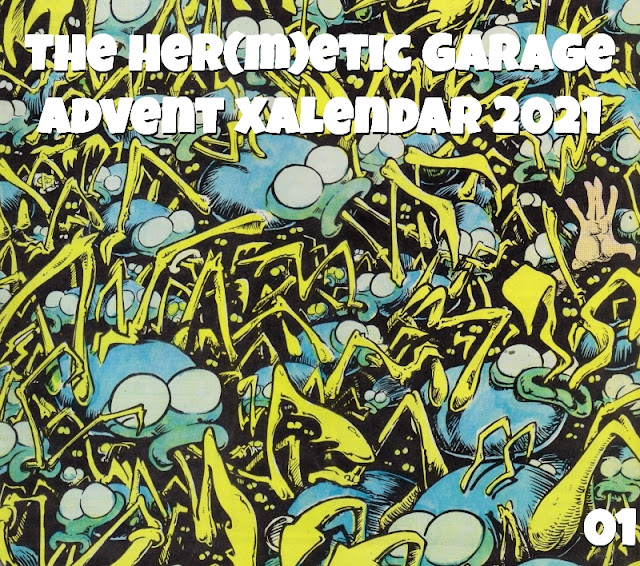Floppy Boot Stomp
•T.U.B.E.
•T.U.B.E.
•T.U.B.E.
•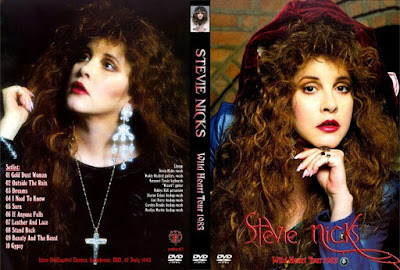T.U.B.E.
•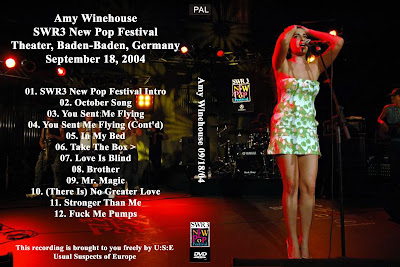T.U.B.E.
•T.U.B.E.
•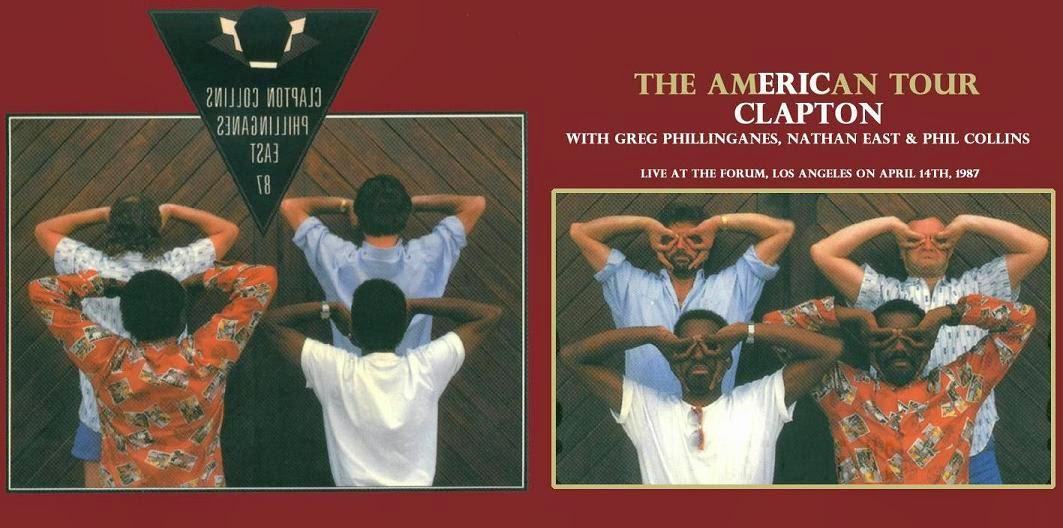T.U.B.E.
•T.U.B.E.
•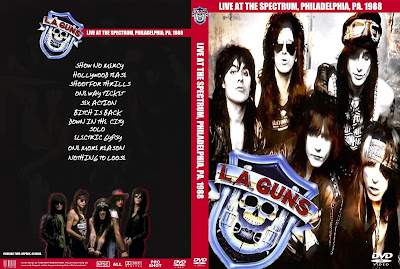T.U.B.E.
•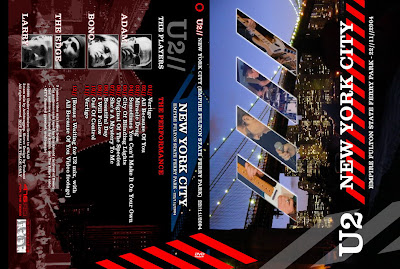T.U.B.E.
•T.U.B.E.
•T.U.B.E.
•T.U.B.E.
•T.U.B.E.
•T.U.B.E.
•Live Bootlegs
•Live Bootlegs
•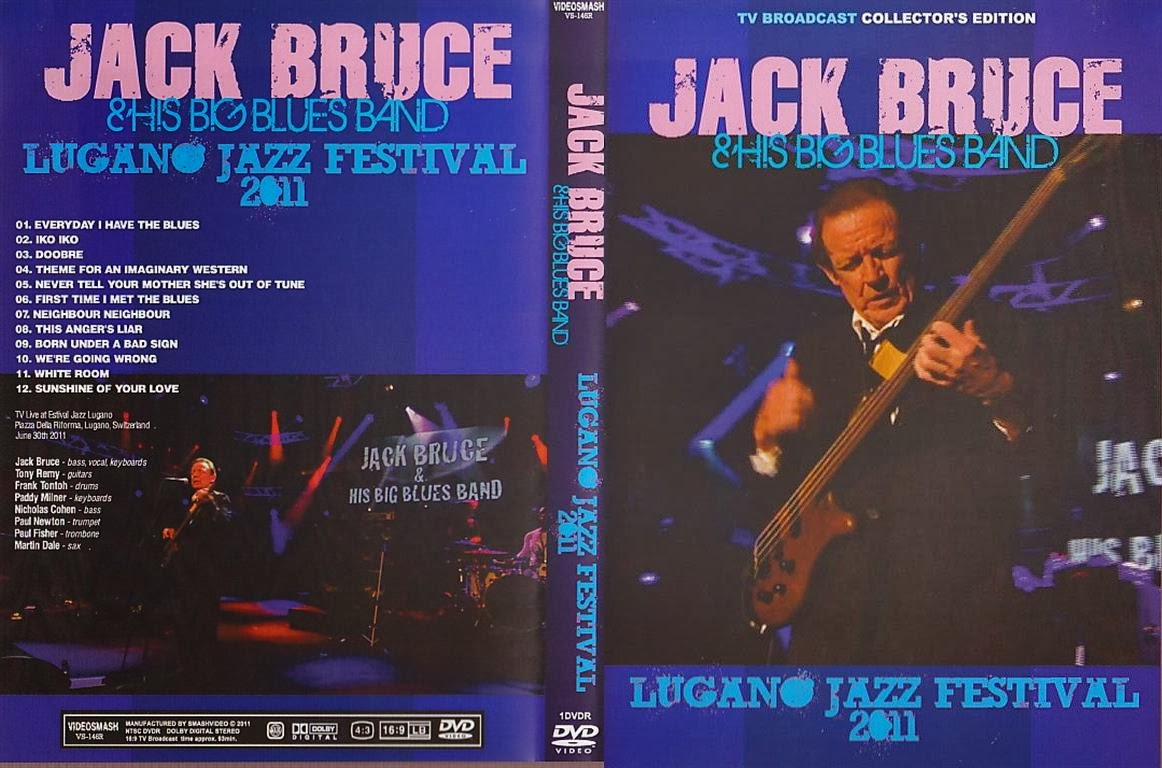T.U.B.E.
•T.U.B.E.
•Floppy Boot Stomp
•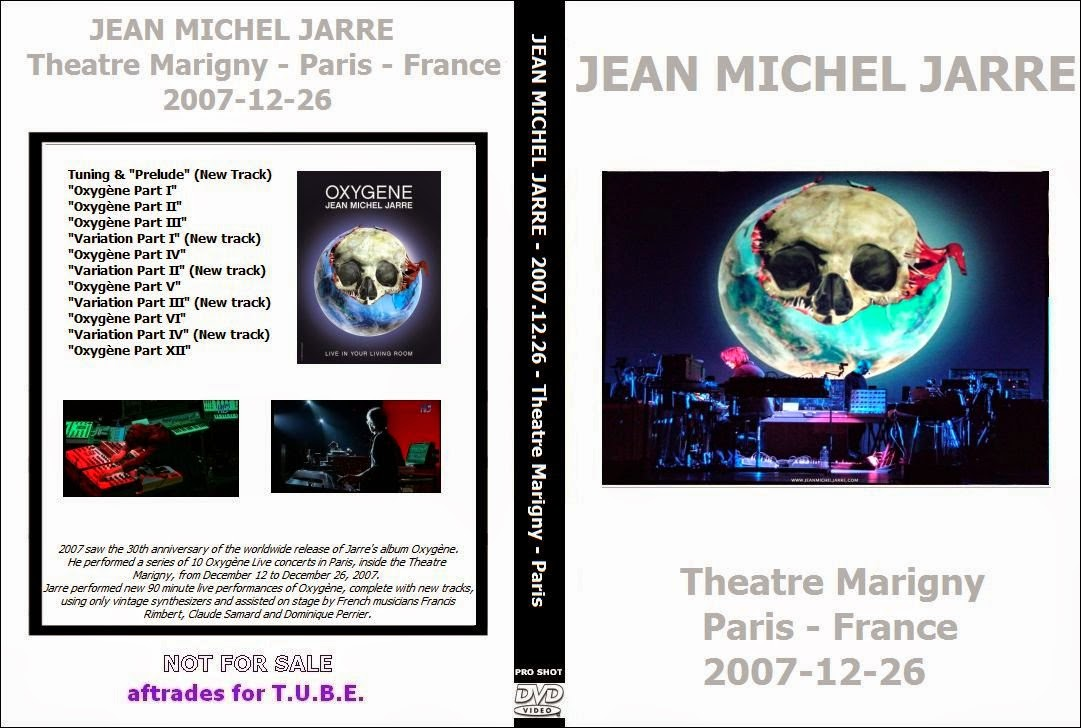T.U.B.E.
1 11 pages | 300 posts | 10 sources